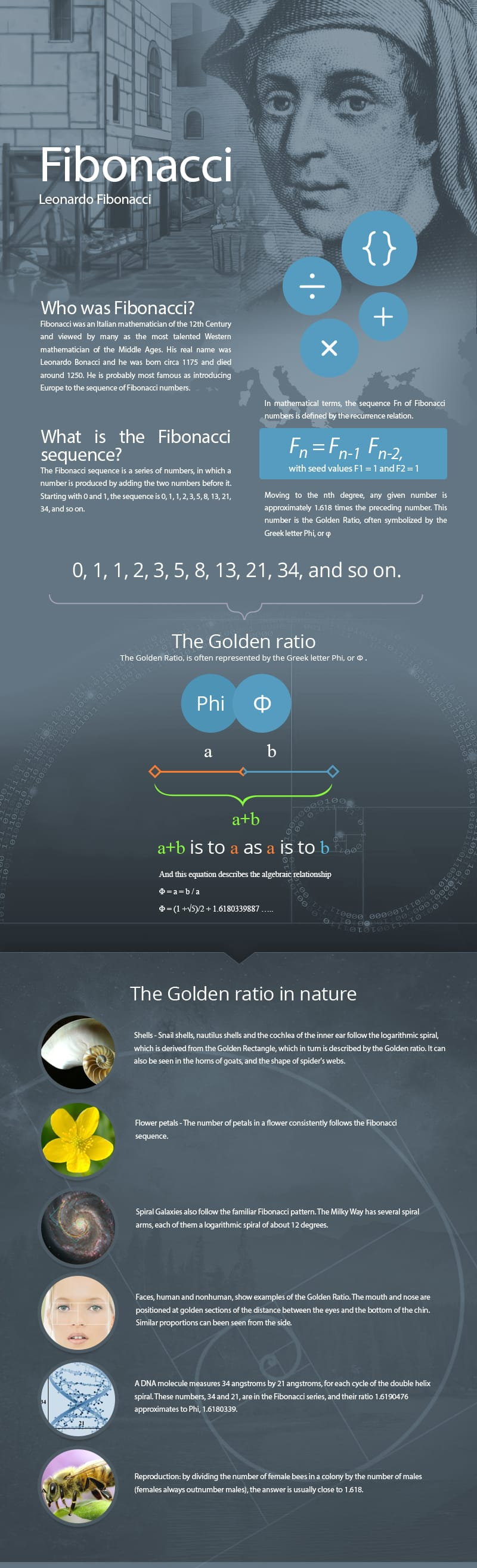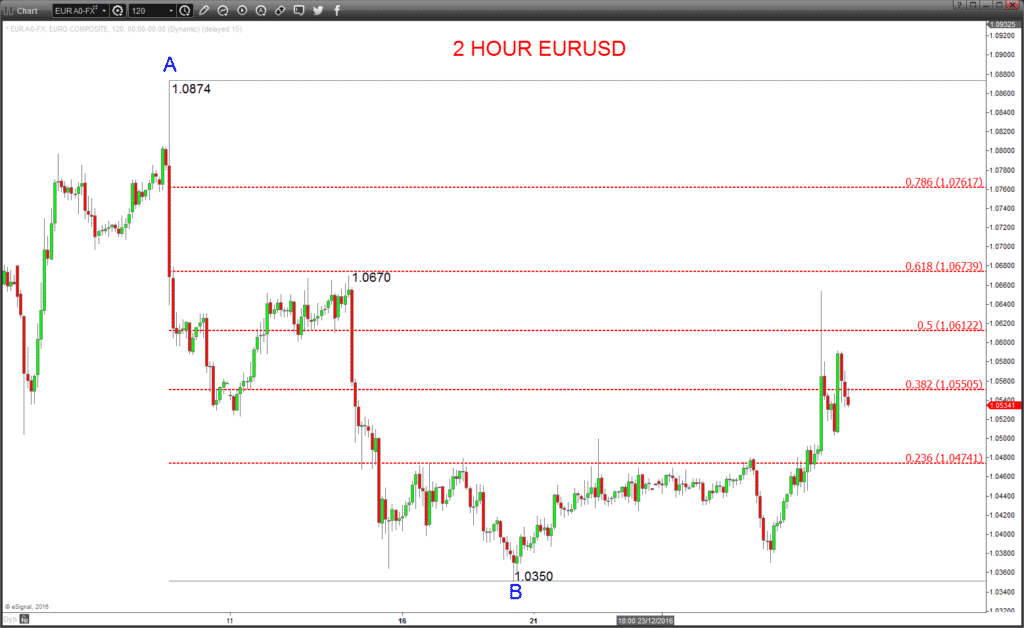The Fibonacci sequence of numbers has been used in trading strategies for many decades now and is a valuable tool in the identification of important levels to monitor when trading markets.

• The historical and mathematical basis of the Fibonacci numbers
• An outline of the various Fibonacci numbers used when trading
• A more detailed examination of how to draw Fibonacci retracements and extensions
• The use of Fibonacci extensions and retracements in trading

## Who was Fibonacci, what is the Fibonacci sequence and how is it connected to the Golden ratio?## Which Fibonacci numbers are used and how are they used in trading?

The main Fibonacci numbers used when trading are:

• 8% – divide any number in the Fibonacci sequence by the next and it tends towards 61.8.
• 2% = 61.8 squared (61.8²) and also derived by dividing any number in the Fibonacci sequence by the number twoplaces to the right.

The second tier of Fibonacci numbers are:

• 6% – divide any number in the Fibonacci sequence by the number three forward and it tends towards 23.6.
• 4% = 100-23.6%.
• 6% = the square root of .618 (√.618).
• 50% is not by definition a Fibonacci number, but is often used by traders.

The third tier of numbers would be:

• 8% is the golden ratio, used mostly for extension levels.
• 2% = the square root of 1.618 (√1.618), also used mostly for extension levels.

There are various ways to use these Fibonacci numbers in trading, including arcs, fans, time zones, retracements and extensions.

In this article, we are going to concentrate on the application of Fibonacci retracement levels and extensions.

## Fibonacci retracements

Fibonacci retracement levels are viewed on a chart as a series of horizontal lines, defining support and resistance.

The technique for establishing the Fibonacci retracement extremes is to decide on the start and finish of any given trend, defined by the timeframe in which the trader is trading. This itself is a subjective decision, does the trend begin at the very low or high of any given trend, or maybe from the first significant high or low from which the trend establishes?

Once the significant high and low of the trend which the trader is analysing is defined, the Fibonacci retracement is applied to the chart, with the 0% and 100% lines drawn at the extremes of the move. From here, 23.6%, 50%, 61.8%, 76.4% and 78.6% retracement levels are extrapolated.

In the EURUSD example in Figure 1, we have defined the 1.0874 high at A as the initial point of the down trend, and the 1.0350 low at B as the current conclusion to this trend phase. We then draw the Fibonacci retracement using points A and B as the extremes and the retracement levels are drawn between these extreme parameters.

Figure 1: 2 Hour EURUSD Chart## Fibonacci extensions

Fibonacci extension (or expansion) levels are drawn similarly to the retracement levels, but rather than identifying just two points from which to draw the retracement, three points are required when drawing a Fibonacci extension.

As with the retracement, the important high and low of a trend are identified as the first two levels from which to draw the extension. Then, after the market has completed an initial retracement of the main trend and established a new swing high or low, this correction high or low is then used as the third point from which the Fibonacci extension levels are then projected.

In the example in Figure 2, the highs and lows of the down trend as seen in Figure 1 are again defined by points A and B. Then the market makes a corrective move up to point C and then attempts to re-energise the underlying down trend. The Fibonacci extension is now drawn from points A and B, then point C, with Fibonacci extension levels then projected below as potential support targets.

Figure 2: 2 Hour EURUSD Chart## The application of Fibonacci retracement and extensions

Primarily, Fibonacci retracement and extension levels can be utilised as support and resistance in order to establish:

Although the Fibonacci levels are important, traders should probably look to identify where the Fibonacci levels coincide with or are close to other important support and resistances.

These might be Pivot levels, impulse highs or lows, swing peaks or troughs, volume/congestion areas or some other defined technical level. If other technical levels are close to or coincide with Fibonacci levels, then these clusters of support or resistance would be viewed as more important. It is then likely that these support/resistance areas would either hold as firm support/ resistance (and produce a shift in direction), or if violated, this would then likely encourage a more impulsive continuation.

Furthermore, some proponents of Fibonacci retracement and extensions use these levels to build very defined trading strategies.

### Steve Miley (The Market Chartist)

Editor in chief

Steve Miley is the Market Chartist and has 29 years of financial market experience and as a seasoned expert now has many responsibilities. He is the founder, Director and Primary Analyst at The Mar... Continued